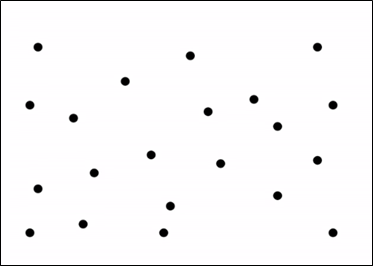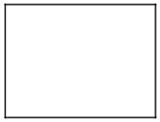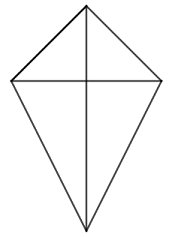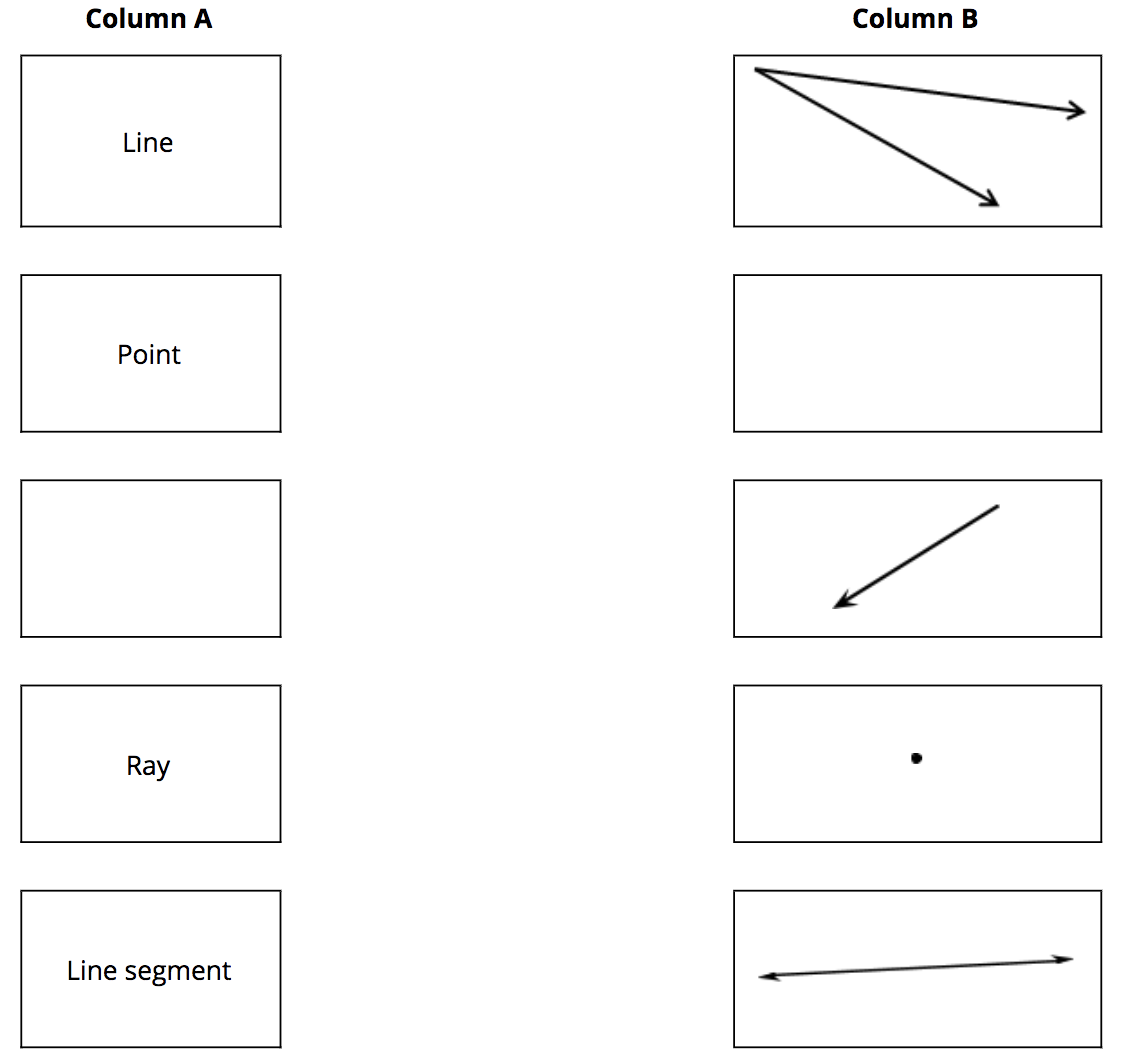# Shapes and Angles

## Objective

Identify and draw points, lines, line segments, rays, and angles.

## Common Core Standards

### Core Standards

?

• 4.G.A.1 — Draw points, lines, line segments, rays, angles (right, acute, obtuse), and perpendicular and parallel lines. Identify these in two-dimensional figures.

• 4.MD.C.5 — Recognize angles as geometric shapes that are formed wherever two rays share a common endpoint, and understand concepts of angle measurement:

?

• 3.G.A.1

## Criteria for Success

?

1. Label geometric objects in order to make it easier for them to be identified, located, and differentiated.
2. Understand that a point is a precise location in space. It has no dimension.
3. Understand that a line is a straight path with no thickness, extending in both directions infinitely. It is one-dimensional. The notation for a line through points $A$ and $B$ is $\overleftrightarrow{AB}$.
4. Understand that a line segment is a part of a line that has two endpoints. It does not extend in either direction infinitely. The notation for a line segment through points $C$ and $D$ is $\overline{CD}$.
5. Understand that a ray is a part of a line that has one endpoint. It extends in one direction infinitely. The notation for a ray through points $E$ and $F$ is $\overset{\rightharpoonup}{EF}$ or $\overrightarrow{EF}$.
6. Understand that an angle is the union of two rays that share a common endpoint. The notation for an angle formed by rays  $\overset{\rightharpoonup}{HG}$and $\overset{\rightharpoonup}{HJ}$ that meet at the point $H$ is $\angle GHJ$.
7. Understand that the vertex of an angle is the point where the two rays meet.
8. Draw points, lines, line segments, rays, and angles using a ruler or straightedge (MP.5).
9. Identify points, lines, line segments, rays, and angles using a ruler or straightedge in two-dimensional figures (MP.2).

## Tips for Teachers

?

In Criteria for Success #5 you will notice there are two different notations for a ray. Students are welcome to use either notation, but for the remainder of this unit we will use the notation $\overrightarrow{AB}$.

### Lesson Materials

• Blank Paper (1 sheet per student)
• Ruler (1 per student) — This can be any tool used to draw a straight line, e.g., a straightedge, a ruler, etc.

#### Fishtank Plus

• Problem Set
• Student Handout Editor
• Vocabulary Package

?

### Problem 1

Silently pick a point from the following image:Write a description of where your point is located.

#### References

Dan Meyer Pick a Point

Pick a Point by Dan Meyer is made available at dy/dan under the CC BY 4.0 license. Accessed Feb. 12, 2018, 1:29 p.m..

### Problem 2

1. Draw two points and label them $A$ and $B$.
2. Draw a line between the two points. Label it $\overleftrightarrow{AB}$.
3. Draw another point that is not on $\overleftrightarrow{AB}$ and label it $C$.
4. Draw a line segment between $A$ and $C$. Label it $\overline{AC}$.
5. Draw another point that is not on $\overleftrightarrow{AB}$ or $\overline{AC}$ and label it $D$.
6. Draw a ray from $B$ to $D$ and label it $\overrightarrow{BD}$.
7. Draw a point that is not on any of the existing objects. Label it $E$.
8. Draw an angle between $D$, $B$, and $E$, and label it $\angle DBE.$

#### References

EngageNY Mathematics Grade 4 Mathematics > Module 4 > Topic A > Lesson 1Concept Development

Grade 4 Mathematics > Module 4 > Topic A > Lesson 1 of the New York State Common Core Mathematics Curriculum from EngageNY and Great Minds. © 2015 Great Minds. Licensed by EngageNY of the New York State Education Department under the CC BY-NC-SA 3.0 US license. Accessed Dec. 2, 2016, 5:15 p.m..

Modified by Fishtank Learning, Inc.

### Problem 3

Identify any points, lines, line segments, rays, and angles in the following figures.#### References

EngageNY Mathematics Grade 4 Mathematics > Module 4 > Topic A > Lesson 1Concept Development

Grade 4 Mathematics > Module 4 > Topic A > Lesson 1 of the New York State Common Core Mathematics Curriculum from EngageNY and Great Minds. © 2015 Great Minds. Licensed by EngageNY of the New York State Education Department under the CC BY-NC-SA 3.0 US license. Accessed Dec. 2, 2016, 5:15 p.m..

Modified by Fishtank Learning, Inc.

## Problem Set & Homework

### Discussion of Problem Set

• Compare your figure to your partner’s for Problem #1. How are they alike? How are they different?
• A point indicates a precise location with no size, only position. Points are infinitely small. Why do we mark them with a dot? Won’t our pencil marks have width? Won’t our pencil marks actually cover many points since the dots we draw have width and points do not?
• Just like a point, a line has no thickness. Can we draw a line that has no thickness, or will we always have to imagine that particular attribute? Why do we draw it on paper with thickness?
• How many corners does a triangle have? A square? A quadrilateral? How does that relate to the number of angles a polygon has?
• How are a ray and a line similar? How are they different?
• How are angles formed? Where have you seen angles before?
• Why is it hard to find real-life examples of lines, points, and rays?
• How does your understanding of a number line connect to this lesson on lines?

?

### Problem 1

Match each term in Column A with a corresponding picture in Column B. One item is missing in each column, which should be filled in to create the remaining pairs.### Problem 2

What makes a line different from a line segment?